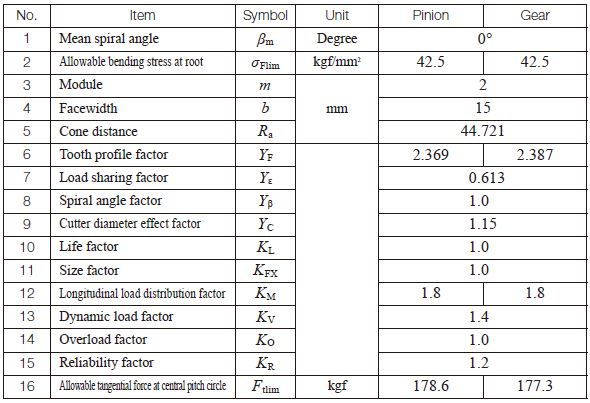TEL : +81 48 254 1744

"Easy" Gear Selection Screen

- Choose a gear by pitch, # of teeth, or bore size.
- 2D/3D Drawings Available
- PDF Catalog Available
- Strength Calculation Available

Go to Gear Selection Screen

CLOSE

# Bending Strength of Bevel Gears

This information is valid for bevel gears which are used for power transmission in general industrial machines. The applicable ranges are:

Transverse module / m / 1.5 – 25mm
Pitch diameter / d0 / 1600mm or less (for straight bevel gears), 1000mm or less (for spiral bevel gears)
Tangential speed / v / 25m/s or less
Rotational speed /n / 3600rpm or less

## (1) Conversion Formulas

In calculating strength, transmitted tangential force at the pitch circle, Ftm (kgf), power, P (kW) , and torque, T (kgf・m) , are the design criteria. Their basic relationships are expressed in the following Equations.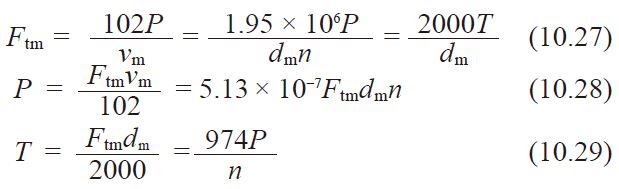Where vm：Tangential speed at the central pitch circle (m/s)
= dmn / 19100

dm：Central pitch diameter (mm)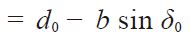## (2) Bending Strength Equations

The tangential force, Ftm, acting at the central pitch circle should be less than the allowable tangential force, Ftmlim, which is based upon the allowable bending stress at the root σFlim.
That is: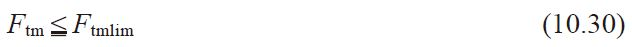The bending stress at the root, σF, which is derived from Ftm should not exceed the allowable bending stress σFlim.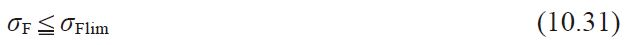The tangential force at the central pitch circle, Ftmlim(kgf) is obtained from Equation (10.32).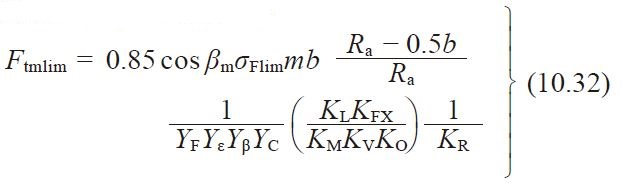Where βm ：Mean spiral angle (degrees)
m ：Transverse module (mm)
Ra ：Cone distance (mm)

The bending strength at the root, σF (kgf/mm2) , is calculated from Equation (10.33).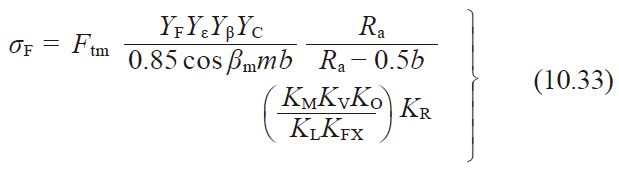## (3) Determination of Various Coefficients

(3)-1 Facewidth b
The term b is defined as the facewidth on the pitch cone. For the meshed pair, the narrower one is used for strength calculations.

(3)-2 Tooth Profile Factor, YF
The tooth profile factor, YF, can be obtained in the following manner: Using Figures 10.8 and 10.9, determine the value of the radial tooth profile factor, YF0. And then, from Figure 10.7 obtain the correction factor, C, for axial shift. Finally, calculate YF by Equation 10.34.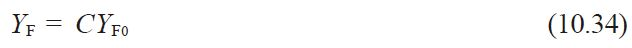Should the bevel gear pair not have any axial shift, the tooth profile factor, YF, is simply YF0.
The equivalent number of teeth, zv, and the profile shift coefficient, x, when using Figures 10.8 and 10.9 is obtainable from Equation (10.35).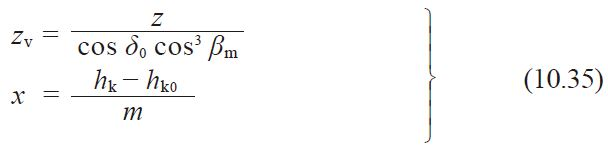Where
m ：Transverse module(mm)
s ：Outer transverse circular tooth thickness(mm)

The axial shift factor, K, is computed from the formula: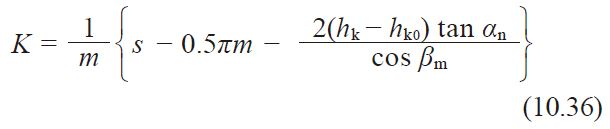Fig.10.7 Correction factor for axial shift, C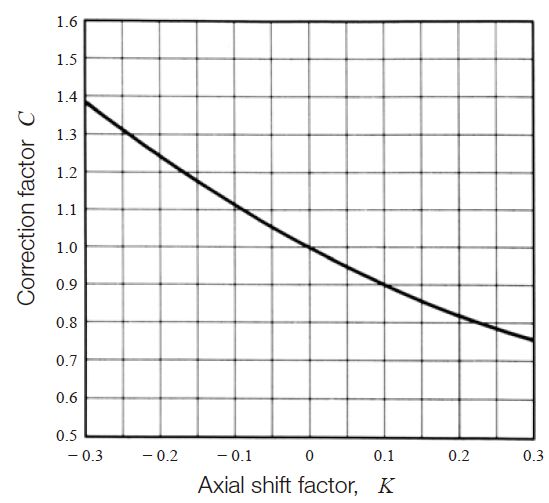Fig.10.8 Tooth profile factor, YF0 (Straight bevel gear)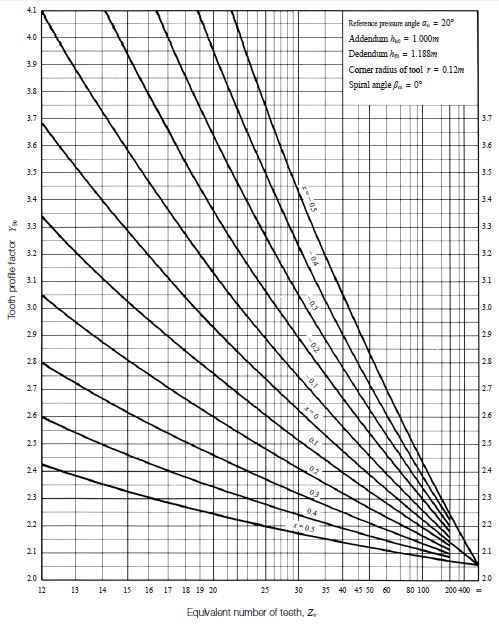Fig.10.9 Tooth profile factor, YF0 (Spiral bevel gear)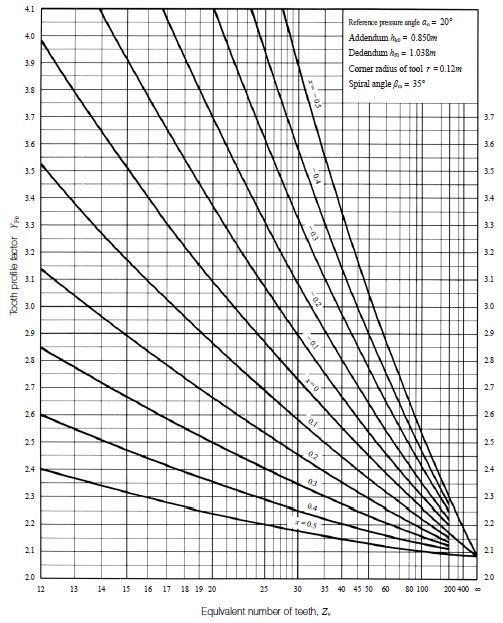Load sharing factor, Yε, is the reciprocal of transverse contact ratio, εα.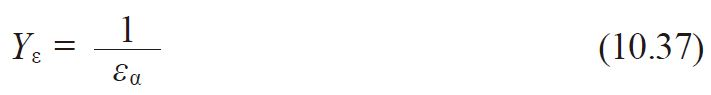The transverse contact ratio, εα, for a straight bevel gear mesh is: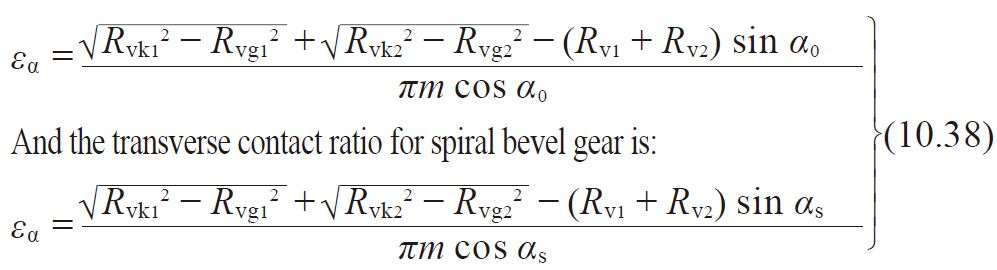See Tables 10.17 – 10.19 for some calculating examples of transverse contact ratio for various bevel gear pairs.

Where:
Rvk :Tip diameter on back cone for equivalent spur gear (mm)
Rvk = Rv + hk = r0secδ0 + hk
Rvg :Reference radius on back cone for equivalent spur gear(mm)
Helical gears = Rv cosα0 = r0secδ0 cosα0
Spiral bevel gears ＝ Rv cosαs = r0secδ0 cosαs
Rv :Back cone distance(mm) = r0secδ0
r0 :Pitch radius(mm) = 0.5z m
α0 :Reference pressure angle (degree)
αs :Mean transverse pressure angle (degree) = tan–1(tanαn/cosβm)
αn :Reference normal pressure angle (degree)

Table 10.17 The transverse contact ratio for Gleason’s straight bevel gear, εα (Σ = 90°、α0 = 20°)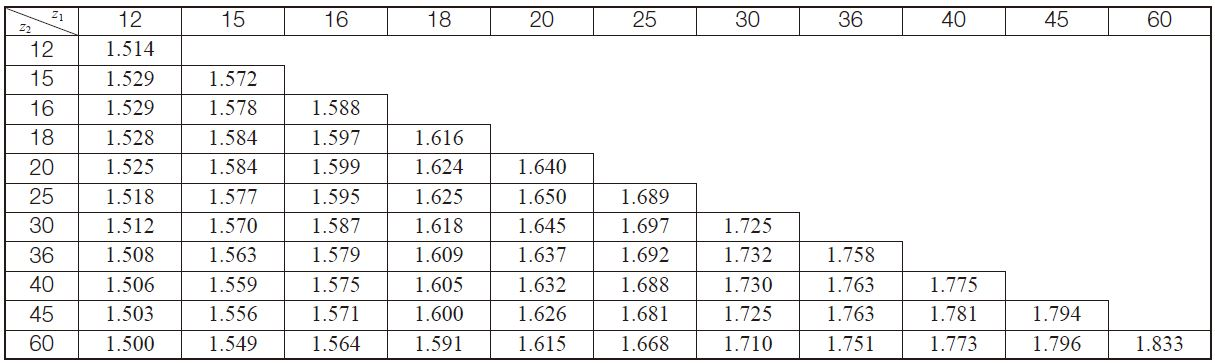Table 10.18 The transverse contact ratio for standard straight bevel gear, εα(Σ = 90°、α0 = 20°)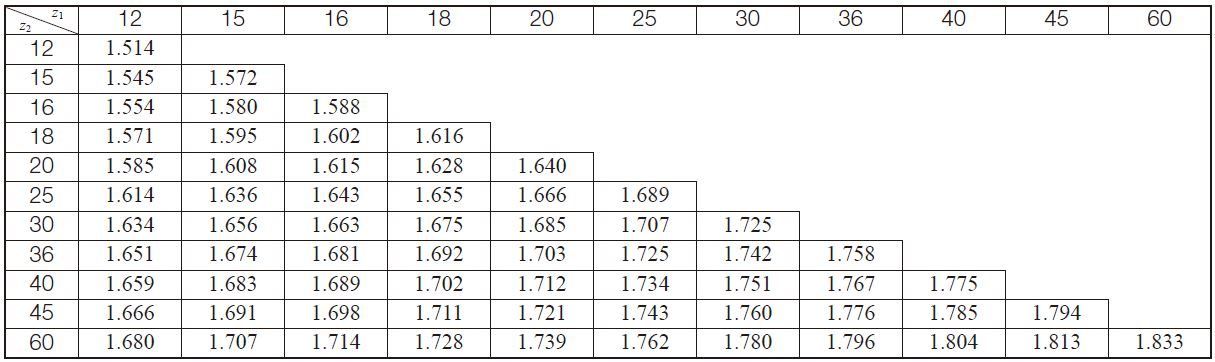Table 10.19 The transverse contact ratio for Gleason’s spiral bevel gear, εα(Σ = 90°、α0 = 20°、βm = 35 deg)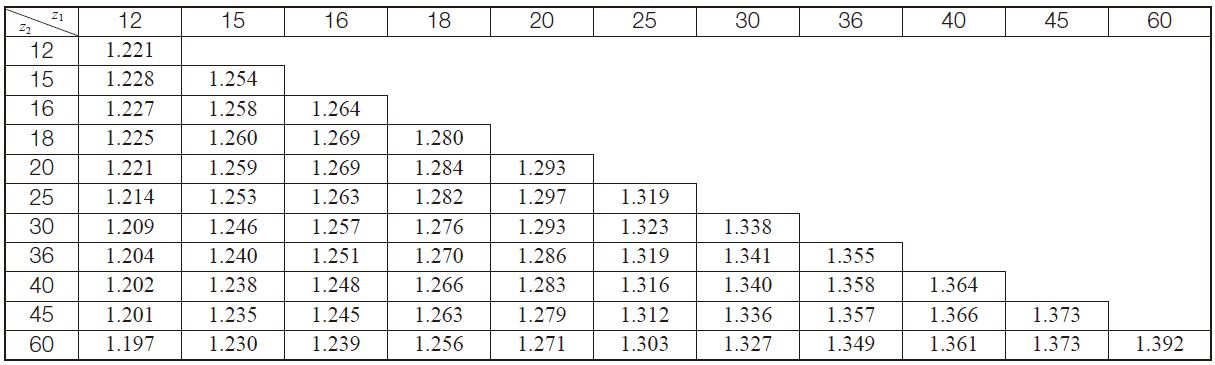(3)-4 Spiral Angle Factor, Yß
The spiral angle factor, Yß, is obtainable from Equation (10.39).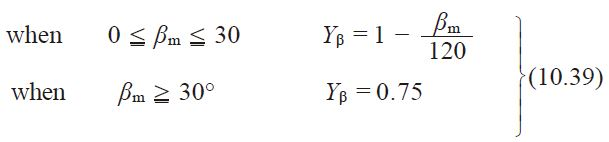(3)-5 Cutter Diameter Effect Factor, YC
The cutter diameter effect factor, YC, can be obtained from Table 10.20 by the value of tooth flank length, b/cos βm (mm), over cutter diameter.
If cutter diameter is not known, assume YC = 1.0.

Table 10.20 Cutter diameter effect factor, YC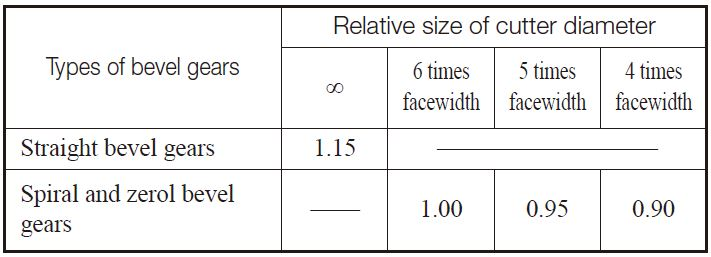(3)-6 Life Factor, KL
The life factor, KL, is obtainable from Table 10.2.

(3)-7 Size Factor of Bending Stress at Root, KFX
The size factor of bending stress at root, KFX, can be obtained from Table 10.21 based on the transverse module, m.

Table 10.21 Size factor for bending strength, KFX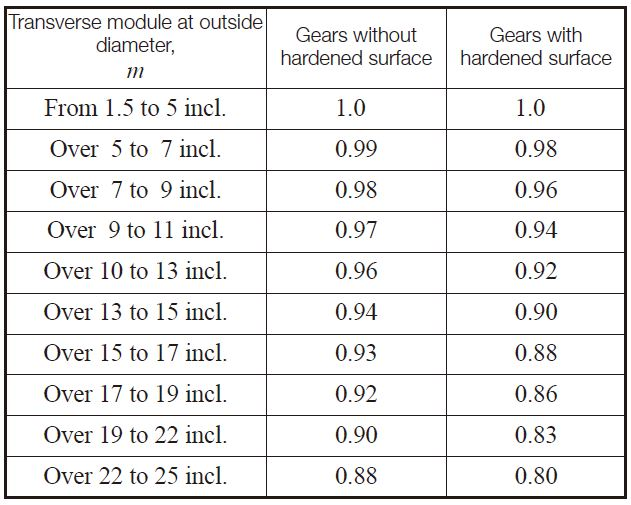(3)-8 Longitudinal Load Distribution Factor, KM
The longitudinal load distribution factor, KM, is obtained from Table 10.22 or Table 10.23.

Table 10.22 Longitudinal load distribution factor, KM for spiral bevel gears, zerol bevel gears and straight bevel gears with crowning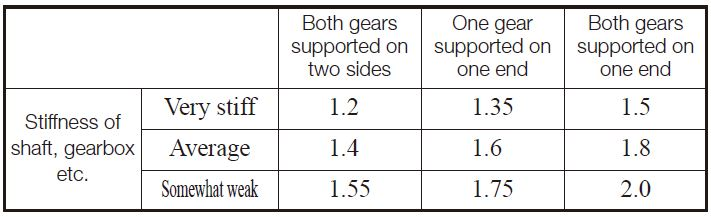Table 10.23 Tooth Flank Load Distribution Factor KM for Straight Bevel Gears without Crowning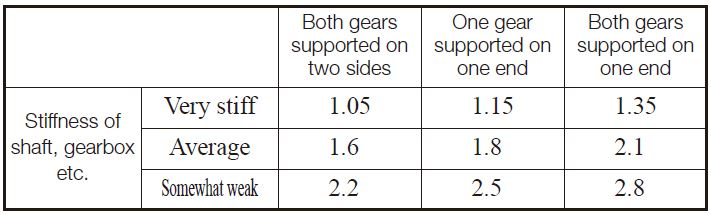Dynamic load factor, KV, is a function of the precision grade of the gear and the tangential speed at the outer pitch circle, as shown in Table 10.24.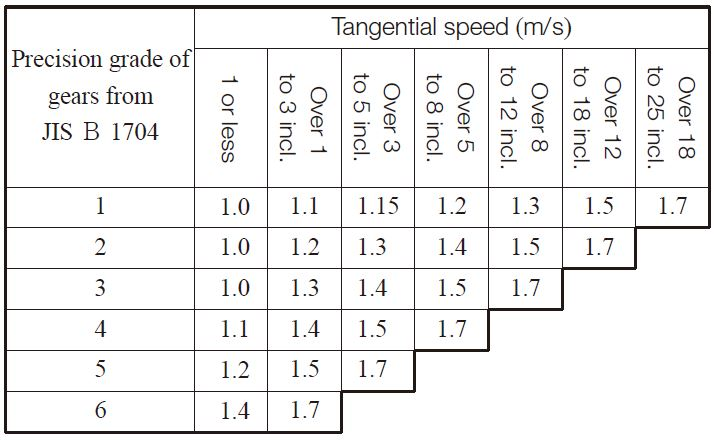The overload factor, KO, can be computed from Equation (10.12) or obtained from Table 10.4, identical to the case of spur and helical gears.

(3)-11 Reliability Factor, KR
The reliability factor, KR, should be assumed to be as follows:
1. General case KR = 1.2
2. When all other factors can be determined accurately: KR = 1.0
3. When all or some of the factors cannot be known with certainty: KR = 1.4

(3)-12 Allowable Bending Stress at Root, σFlim
The allowable bending stress at the root is obtained by a bending strength calculation for spur and helical gears as shown at < (3)-10 >.

## (4) Example of Calculation

Gleason straight bevel gear design details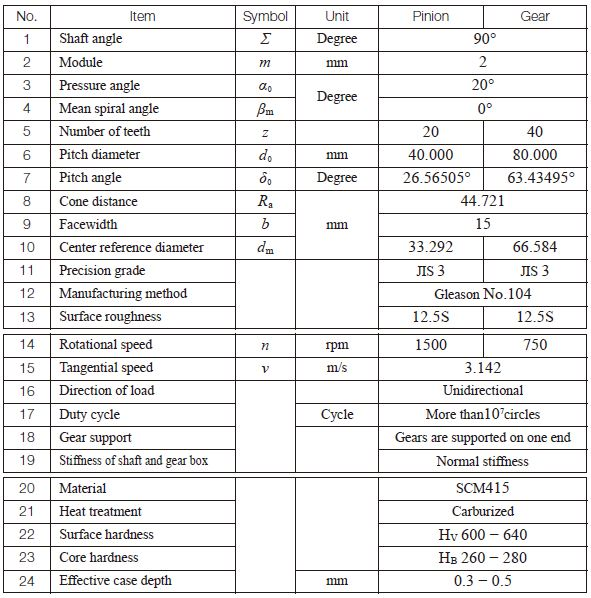Bending strength factors for Gleason straight bevel gear